Updating search results...

# 14 Results

View
Selected filters:
• MCCRS.Math.Content.6.EE.A.4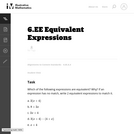Unrestricted Use
CC BY
Rating
0.0 stars

In this problem students must transform expressions using the distributive, commutative and associative properties to decide which expressions are equivalent.

Subject:
Mathematics
Material Type:
Activity/Lab
Provider:
Illustrative Mathematics
Provider Set:
Illustrative Mathematics
Author:
Illustrative Mathematics
05/01/2012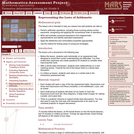Only Sharing Permitted
CC BY-NC-ND
Rating
0.0 stars

This lesson unit is intended to help you assess how well students are able to: Perform arithmetic operations, including those involving whole-number exponents, recognizing and applying the conventional order of operations; Write and evaluate numerical expressions from diagrammatic representations and be able to identify equivalent expressions; apply the distributive and commutative properties appropriately; and use the method for finding areas of compound rectangles.

Subject:
Geometry
Mathematics
Material Type:
Assessment
Lesson Plan
Provider:
Shell Center for Mathematical Education
Provider Set:
Mathematics Assessment Project (MAP)
04/26/2013Conditional Remix & Share Permitted
CC BY-NC
Rating
0.0 stars
Rating
0.0 stars
Subject:
Mathematics
Material Type:
Full Course
Provider:
Pearson
10/06/2016Conditional Remix & Share Permitted
CC BY-NC
Rating
0.0 stars

Expressions

Type of Unit: Concept

Prior Knowledge

Students should be able to:

Write and evaluate simple expressions that record calculations with numbers.
Use parentheses, brackets, or braces in numerical expressions and evaluate expressions with these symbols.
Interpret numerical expressions without evaluating them.

Lesson Flow

Students learn to write and evaluate numerical expressions involving the four basic arithmetic operations and whole-number exponents. In specific contexts, they create and interpret numerical expressions and evaluate them. Then students move on to algebraic expressions, in which letters stand for numbers. In specific contexts, students simplify algebraic expressions and evaluate them for given values of the variables. Students learn about and use the vocabulary of algebraic expressions. Then they identify equivalent expressions and apply properties of operations, such as the distributive property, to generate equivalent expressions. Finally, students use geometric models to explore greatest common factors and least common multiples.

Subject:
Algebra
Mathematics
Material Type:
Unit of Study
Provider:
PearsonConditional Remix & Share Permitted
CC BY-NC
Rating
0.0 stars

Students critique the work of other students and revise their own work based on feedback from the teacher and peers.Key ConceptsConcepts from previous lessons are integrated into this unit task: rewriting expressions, using parentheses, and using the distributive property. Students apply their knowledge, review their work, and make revisions based on feedback from you and their peers. This process creates a deeper understanding of the concepts.Goals and Learning ObjectivesApply knowledge of expressions to correct the work of other students.Track and review the choice of strategy when problem solving.

Subject:
Mathematics
Material Type:
Lesson Plan
09/21/2015Conditional Remix & Share Permitted
CC BY-NC
Rating
0.0 stars

Students express the lengths of trains as algebraic expressions and then substitute numbers for letters to find the actual lengths of the trains.Key ConceptsAn algebraic expression can be written to represent a problem situation. More than one algebraic expression may represent the same problem situation. These algebraic expressions have the same value and are equivalent.To evaluate an algebraic expression, a specific value for each variable is substituted in the expression, and then all the calculations are completed using the order of operations to get a single value.Goals and Learning ObjectivesEvaluate expressions for the given values of the variables.

Subject:
Algebra
Material Type:
Lesson Plan
09/21/2015Conditional Remix & Share Permitted
CC BY-NC
Rating
0.0 stars

Students represent problem situations using expressions and then evaluate the expressions for the given values of the variables.Key ConceptsAn algebraic expression can be written to represent a problem situation.To evaluate an algebraic expression, a specific value for each variable is substituted in the expression, and then all the calculations are completed using the order of operations to get a single value.Goals and Learning ObjectivesDevelop fluency in writing expressions to represent situations and in evaluating the expressions for given values.

Subject:
Algebra
Material Type:
Lesson Plan
09/21/2015Conditional Remix & Share Permitted
CC BY-NC
Rating
0.0 stars

Getting Started

Type of Unit: Introduction

Prior Knowledge

Students should be able to:

Solve and write numerical equations for whole number addition, subtraction, multiplication, and division problems.
Use parentheses to evaluate numerical expressions.
Identify and use the properties of operations.

Lesson Flow

In this unit, students are introduced to the rituals and routines that build a successful classroom math community and they are introduced to the basic features of the digital course that they will use throughout the year.

An introductory card sort activity matches students with their partner for the week. Then over the course of the week, students learn about the lesson routines: Opening, Work Time, Ways of Thinking, Apply the Learning, Summary of the Math, and Reflection. Students learn how to present their work to the class, the importance of taking responsibility for their own learning, and how to effectively participate in the classroom math community.

Students then work on Gallery problems to further explore the program’s technology resources and tools and learn how to organize their work.

The mathematical work of the unit focuses on numerical expressions, including card sort activities in which students identify equivalent expressions and match an expression card to a word card that describes its meaning. Students use the properties of operations to identify equivalent expressions and to find unknown values in equations.

Subject:
Mathematics
Material Type:
Unit of Study
Provider:
PearsonConditional Remix & Share Permitted
CC BY-NC-SA
Rating
0.0 stars

Students are introduced to classroom routines and expectations, and complete a full mathematics lesson. The class discusses how to clearly present work to classmates. Partner work is modeled, and partners then work to match numerical expressions to corresponding word descriptions. Students read and discuss a summary of the math in the lesson, and then write a reflection about their thoughts.Key ConceptsStudents match a numerical expression to its corresponding description in words. Students interpret parentheses and brackets in numerical expressions and they construct viable arguments and critique the reasoning of others. Students learn to use the exponent 2 to represent squaring.Goals and Learning ObjectivesDescribe the classroom routines and expectations.Consider how to present work clearly to classmates.Collaborate with a partner.Critique a partner&rsquo;s reasoning.Connect a numerical expression to its corresponding word description.Learn to use an exponent of 2 to represent squaring.

Subject:
Algebra
Material Type:
Lesson Plan
Author:
Benjamin White
08/23/2018Conditional Remix & Share Permitted
CC BY-NC
Rating
0.0 stars

The class reviews the properties of operations. The use of &ldquo;ask myself&rdquo; questions to make sense of problems and persevere is modeled. Students review things to do when they feel stuck on a problem. Finally, students use the properties of operations to evaluate expressions.Key ConceptsStudents use the properties of operations to justify whether two expressions are equivalent.Goals and Learning ObjectivesTo start to work on a problem, make sense of the problem by using &ldquo;ask myself&rdquo; questions.Persevere in solving a problem even when feeling stuck.Use the properties of operations to evaluate expressions.

Subject:
Algebra
Material Type:
Lesson Plan
09/21/2015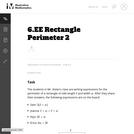Unrestricted Use
CC BY
Rating
0.0 stars

This task is a natural follow up for task Rectangle Perimeter 1. After thinking about and using one specific expression for the perimeter of a rectangle, students now extend their thinking to equivalent expressions for the same quantity.

Subject:
Mathematics
Material Type:
Activity/Lab
Provider:
Illustrative Mathematics
Provider Set:
Illustrative Mathematics
Author:
Illustrative Mathematics
05/01/2012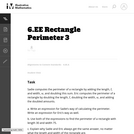Unrestricted Use
CC BY
Rating
0.0 stars

The purpose of this task is to ask students to write expressions and to consider what it means for two expressions to be equivalent.

Subject:
Mathematics
Material Type:
Activity/Lab
Provider:
Illustrative Mathematics
Provider Set:
Illustrative Mathematics
Author:
Illustrative Mathematics
08/07/2012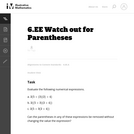Unrestricted Use
CC BY
Rating
0.0 stars

This problem asks the student to evaluate three numerical expressions that contain the same integers yet have differing results due to placement of parentheses.

Subject:
Mathematics
Material Type:
Activity/Lab
Provider:
Illustrative Mathematics
Provider Set:
Illustrative Mathematics
Author:
Illustrative Mathematics